# Overlapping triangle drill

14 Jan 2015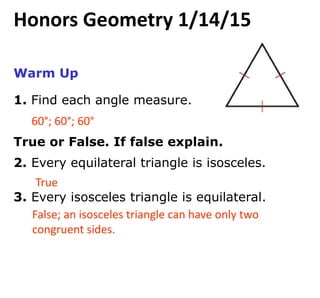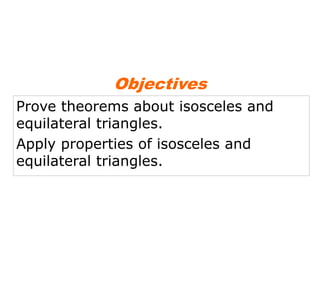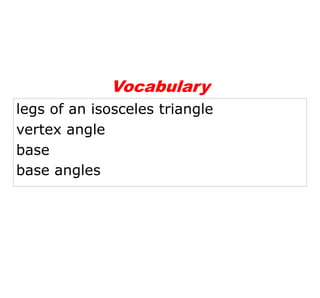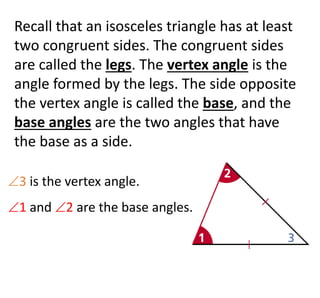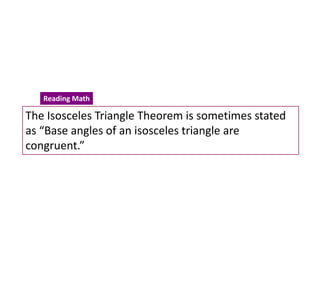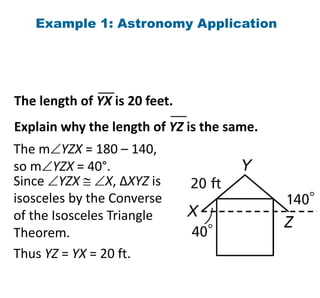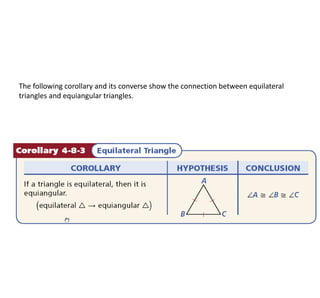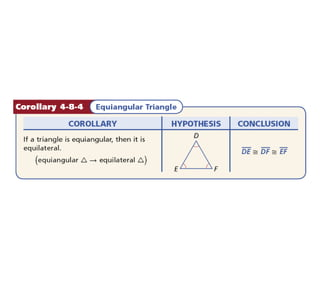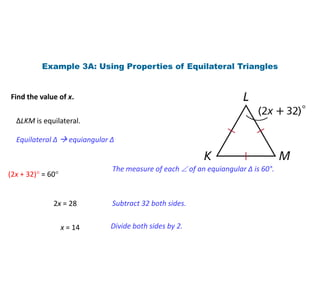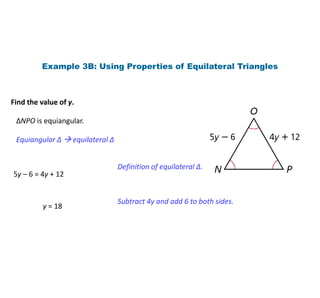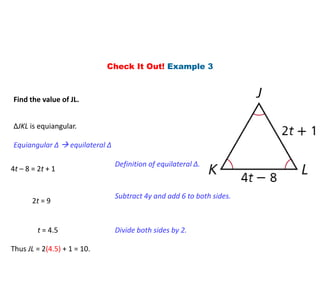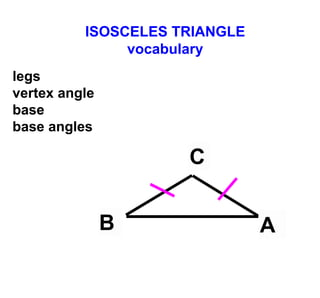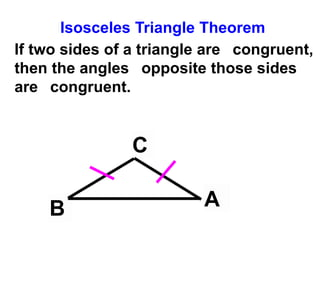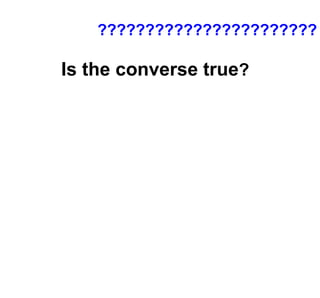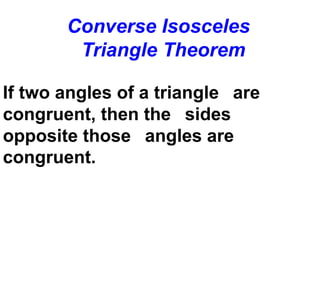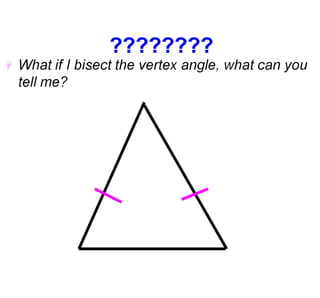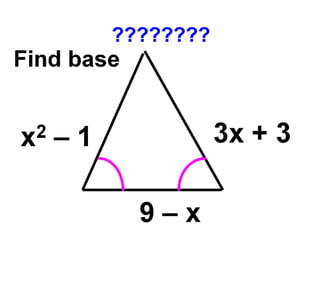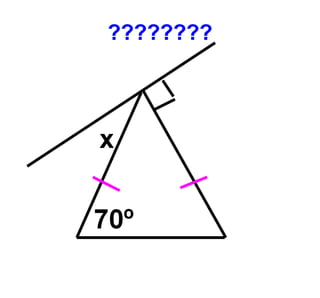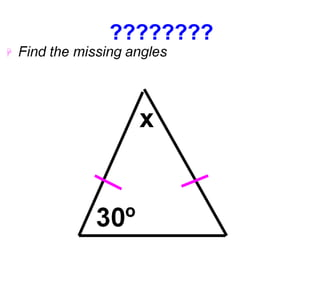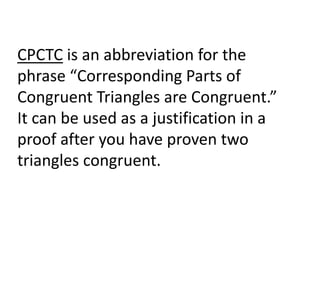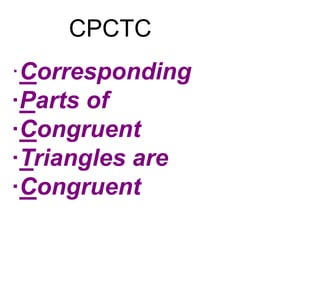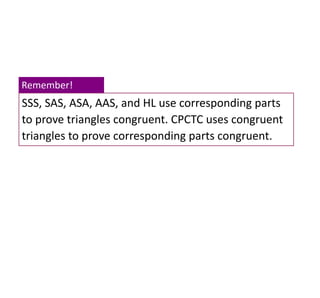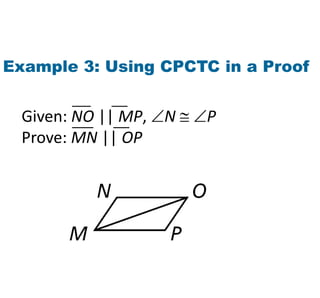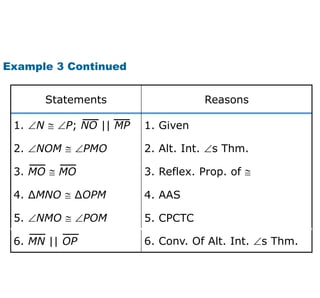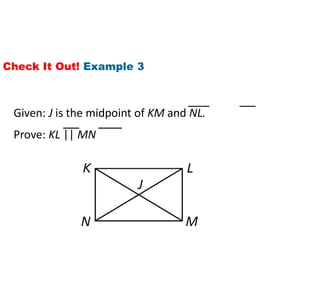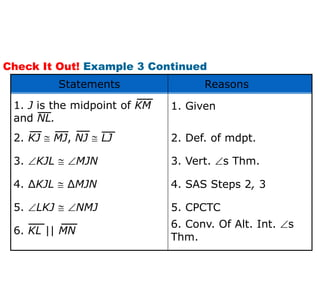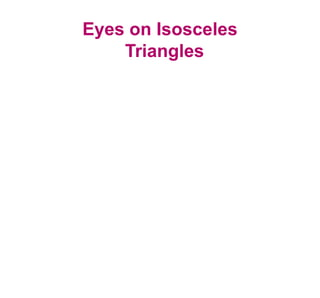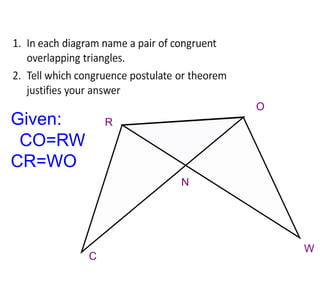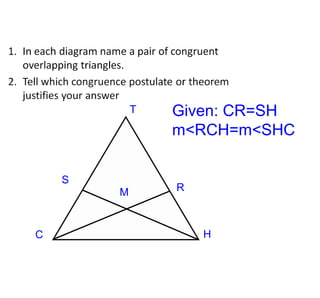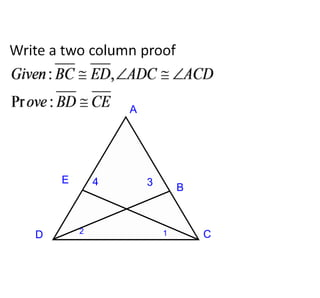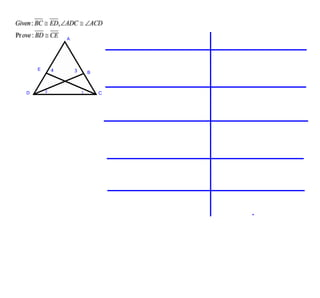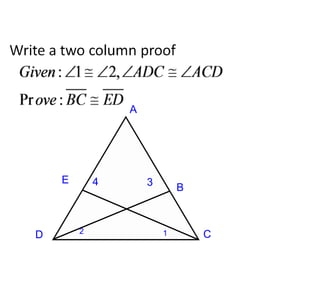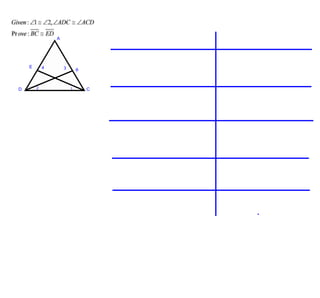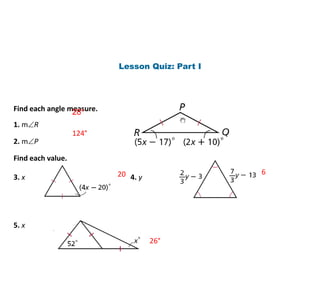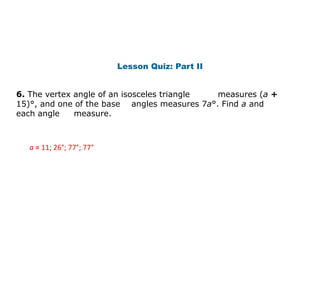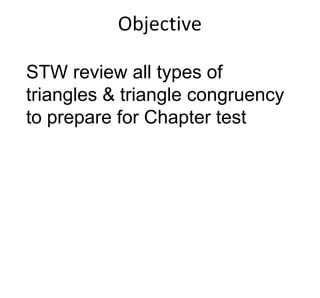1 sur 38

### Overlapping triangle drill

• 1. Warm Up 1. Find each angle measure. True or False. If false explain. 2. Every equilateral triangle is isosceles. 3. Every isosceles triangle is equilateral. 60°; 60°; 60° True False; an isosceles triangle can have only two congruent sides. Honors Geometry 1/14/15
• 3. Prove theorems about isosceles and equilateral triangles. Apply properties of isosceles and equilateral triangles. Objectives
• 4. legs of an isosceles triangle vertex angle base base angles Vocabulary
• 5. Recall that an isosceles triangle has at least two congruent sides. The congruent sides are called the legs. The vertex angle is the angle formed by the legs. The side opposite the vertex angle is called the base, and the base angles are the two angles that have the base as a side. 3 is the vertex angle. 1 and 2 are the base angles.
• 7. The Isosceles Triangle Theorem is sometimes stated as “Base angles of an isosceles triangle are congruent.” Reading Math
• 8. Example 1: Astronomy Application The length of YX is 20 feet. Explain why the length of YZ is the same. Since YZX  X, ∆XYZ is isosceles by the Converse of the Isosceles Triangle Theorem. The mYZX = 180 – 140, so mYZX = 40°. Thus YZ = YX = 20 ft.
• 9. The following corollary and its converse show the connection between equilateral triangles and equiangular triangles.
• 11. Example 3A: Using Properties of Equilateral Triangles Find the value of x. ∆LKM is equilateral. (2x + 32) = 60 The measure of each  of an equiangular ∆ is 60°. 2x = 28 Subtract 32 both sides. x = 14 Divide both sides by 2. Equilateral ∆  equiangular ∆
• 12. Example 3B: Using Properties of Equilateral Triangles Find the value of y. ∆NPO is equiangular. Equiangular ∆  equilateral ∆ 5y – 6 = 4y + 12 Definition of equilateral ∆. y = 18 Subtract 4y and add 6 to both sides.
• 13. Check It Out! Example 3 Find the value of JL. ∆JKL is equiangular. Equiangular ∆  equilateral ∆ 4t – 8 = 2t + 1 Definition of equilateral ∆. 2t = 9 Subtract 4y and add 6 to both sides. t = 4.5 Divide both sides by 2. Thus JL = 2(4.5) + 1 = 10.
• 15. C Isosceles Triangle Theorem If two sides of a triangle are congruent, then the angles opposite those sides are congruent. B A
• 17. Converse Isosceles Triangle Theorem If two angles of a triangle are congruent, then the sides opposite those angles are congruent.
• 22. CPCTC is an abbreviation for the phrase “Corresponding Parts of Congruent Triangles are Congruent.” It can be used as a justification in a proof after you have proven two triangles congruent.
• 24. SSS, SAS, ASA, AAS, and HL use corresponding parts to prove triangles congruent. CPCTC uses congruent triangles to prove corresponding parts congruent. Remember!
• 25. Example 3: Using CPCTC in a Proof Prove: MN || OP Given: NO || MP, N  P
• 26. 5. CPCTC5. NMO  POM 6. Conv. Of Alt. Int. s Thm. 4. AAS4. ∆MNO  ∆OPM 3. Reflex. Prop. of  2. Alt. Int. s Thm.2. NOM  PMO 1. Given ReasonsStatements 3. MO  MO 6. MN || OP 1. N  P; NO || MP Example 3 Continued
• 27. Check It Out! Example 3 Prove: KL || MN Given: J is the midpoint of KM and NL.
• 28. Check It Out! Example 3 Continued 5. CPCTC5. LKJ  NMJ 6. Conv. Of Alt. Int. s Thm. 4. SAS Steps 2, 34. ∆KJL  ∆MJN 3. Vert. s Thm.3. KJL  MJN 2. Def. of mdpt. 1. Given ReasonsStatements 6. KL || MN 1. J is the midpoint of KM and NL. 2. KJ  MJ, NJ  LJ
• 36. Lesson Quiz: Part I Find each angle measure. 1. mR 2. mP Find each value. 3. x 4. y 5. x 124° 28° 20 6 26°
• 37. Lesson Quiz: Part II 6. The vertex angle of an isosceles triangle measures (a + 15)°, and one of the base angles measures 7a°. Find a and each angle measure. a = 11; 26°; 77°; 77°
• 38. STW review all types of triangles & triangle congruency to prepare for Chapter test